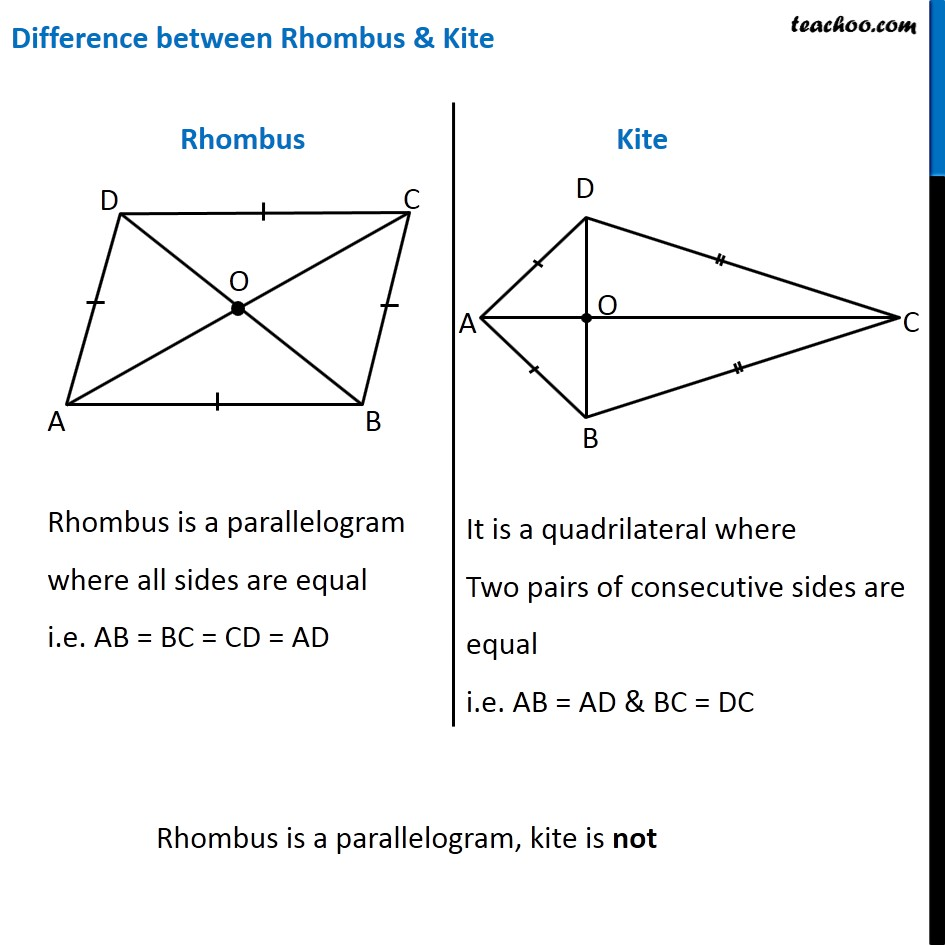Rhombus, Rectangle, Square

Chapter 3 Class 8 Understanding Quadrilaterals
Concept wiseLearn in your speed, with individual attention - Teachoo Maths 1-on-1 Class

### Transcript

Difference between Rhombus & Kite Rhombus Kite Rhombus is a parallelogram where all sides are equal i.e. AB = BC = CD = AD It is a quadrilateral where Two pairs of consecutive sides are equal i.e. AB = AD & BC = DC Rhombus is a parallelogram, kite is not